# Check Against Reference

Check that model signal tracks reference signal during simulation

•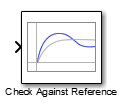Libraries:
Simulink Design Optimization / Model Verification
Simulink Design Optimization / Signal Constraints

## Description

Check that a signal remains within the tolerance bounds of a reference signal during simulation.

• If the signal satisfies all bounds, the block does nothing.

• If the signal does not satisfy a bound, a warning message appears in the MATLAB® command window. You can also specify that the block:

• Evaluate a MATLAB expression.

• Stop the simulation and return an error message.

During simulation, the block can also return a logical assertion signal.

• If the signal satisfies all bounds, the assertion signal is true (`1`).

• If the signal does not satisfy a bound, the assertion signal is false (`0`).

You can add Check Against Reference blocks to multiple signals to check that they track reference signals. You can also plot the reference signal on a time plot to graphically verify the signal tracking.

This block and the other blocks in the Model Verification library test that a signal remains within specified time-domain characteristic bounds. When a model does not violate any bound, you can disable the block by clearing the assertion option. If you modify the model, you can re-enable assertion to ensure that your changes do not cause the model to violate a bound.

If the signal does not satisfy the bounds, you can optimize the model parameters to satisfy the bounds. If you have Simulink® Control Design™ software, you can add frequency-domain bounds, such as the Bode magnitude, and optimize the model response to satisfy both time-domain and frequency-domain requirements.

The block can be used in all simulation modes for signal monitoring, but only in `Normal` or `Accelerator` simulation mode for response optimization.

## Ports

### Input

expand all

Signal to check against the reference signal specified in Amplitudes and Times during simulation.

Data Types: `single` | `double` | `int8` | `int16` | `int32` | `int64` | `uint8` | `uint16` | `uint32` | `uint64` | `fixed point` | `enumerated` | `bus`

### Output

expand all

Output signal indicating if the input signal is within bounds of the specified assertion signal. At each time step, the output is returned as one of the following:

• True (`1`) if the assertion succeeds, that is, the input signal satisfies all bounds

• False (`0`) if the assertion fails, that is, the signal violates a bound

The data type of the output signal is double by default. To set the output data type as Boolean for all blocks that generate logic signals in your Simulink model, select Implement logic signals as Boolean data in Configuration Parameters.

#### Dependencies

To enable this port, on the Assertion tab, select the Output assertion signal parameter.

Data Types: `Boolean` | `double`

## Parameters

expand all

Use the plot to view the following.

• System characteristics and signals computed during simulation — Click this button before you simulate the model to view the system characteristics or signal.

You can display additional characteristics, such as the peak response time, by right-clicking the plot and selecting Characteristics.

• Bounds — You can specify bounds on the Bounds tab or by right-clicking the plot and selecting Bounds > New Bound.

You can modify bounds by dragging the bound segment or by right-clicking the plot and selecting Bounds > Edit Bound. Before you simulate the model, click to update the bound value in the block parameters.

Typical tasks that you perform in the plot window include:

• Opening the Block Parameters dialog box by clicking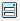or selecting Edit.

• Finding the block that the plot window corresponds to by clicking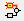or selecting View > Highlight Simulink Block. This action makes the model window active and highlights the block.

• Simulating the model by clicking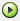. This action also linearizes the portion of the model between the specified linearization input and output.

• Adding a legend on the linear system characteristic plot by clicking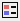.

Open the plot window instead of the block parameters when you double-click the block in the Simulink model.

Use this parameter if you prefer to open and perform tasks, such as adding or modifying bounds, in the plot window instead of the block parameters. If you want to access the block parameters from the plot window, select Edit or click.

#### Programmatic Use

 Parameter: `LaunchViewOnOpen` Type: character vector Value: `'on'` | `'off'` Default: `'off'`

Open the Response Optimizer to optimize the model response to meet design requirements specified on the Bounds tab.

### Bounds Tab

Check that the input signal to the block tracks the reference signal specified in Amplitudes and Times during simulation. The software displays a warning if the signal does not track the reference signal.

• When you disable this parameter, the software stops checking that the input signal tracks the reference during simulation.

• To view only the reference signal on the plot, disable Enable assertion.

The reference signal appears on a time plot if you select Show plot, as shown in the figure.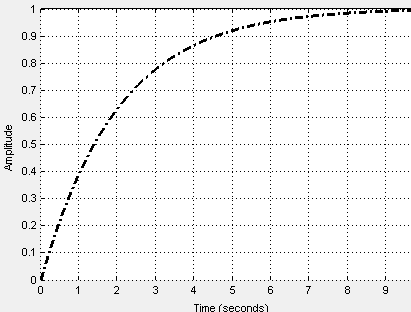#### Dependencies

To use this parameter, select Enable assertion in block parameters. When Enable assertion is disabled, the software does not use the reference signal for assertion, but it continues to appear on the plot.

#### Programmatic Use

 Parameter: `EnableReferenceBound` Type: character vector Value: `'on'` | `'off'` Default: `'on'`

Time vector for the reference signal in seconds, specified as a monotonically increasing vector of positive values. You can also specify a MATLAB expression that evaluates to a vector. Specify the corresponding amplitudes using the Amplitudes parameter. The Times vector must have the same dimension as the Amplitudes vector.

#### Programmatic Use

 Parameter: `ReferenceTimes` Type: vector Value: vector of positive values | MATLAB expression Default: `linspace(0,10)`

Amplitude of the reference signal, specified as a real and finite vector, corresponding to the time vector specified in Times. You can also specify a MATLAB expression that evaluates to a vector. The Amplitudes vector must have the same dimension as the Times (seconds) vector.

#### Programmatic Use

 Parameter: `ReferenceAmplitudes` Type: vector Value: vector | MATLAB expression Default: `1-exp(-linspace(0,10)/2)`

Absolute tolerance used to determine bounds as the input signal approaches the reference signal, specified as a positive real scalar. During simulation, the signal must remain within upper and lower limits respective to the reference signal given by

yu = (1 + RelTol)yr + AbsTol

yl = (1 – RelTol)yrAbsTol

Here:

• yr is the value of the reference at a certain time.

• yu is the upper tolerance bound corresponding to that time point.

• yl is the lower tolerance bound corresponding to that time point.

• RelTol sets the relative tolerance between input and reference signal amplitudes at each time step. To set RelTol, use the Relative tolerance parameter.

• AbsTol sets the absolute tolerance between input and reference signal amplitudes at each time step. To set AbsTol, use the Absolute tolerance parameter.

The block asserts if the signal violates these limits.

#### Programmatic Use

 Parameter: `AbsTolerance` Type: scalar Value: positive real scalar Default: `eps^(1/3)`

Relative tolerance used to determine bounds as the input signal approaches the reference signal, specified as a positive real scalar. During simulation, the signal must remain within upper and lower limits respective to the reference signal given by

yu = (1 + RelTol)yr + AbsTol

yl = (1 – RelTol)yrAbsTol

Here:

• yr is the value of the reference at a certain time.

• yu is the upper tolerance bound.

• yl is the lower tolerance bound.

• RelTol sets the relative tolerance between input and reference signal amplitudes at each time step. To set RelTol, use the Relative tolerance parameter.

• AbsTol sets the absolute tolerance between input and reference signal amplitudes at each time step. To set AbsTol, use the Absolute tolerance parameter.

The block asserts if the signal violates these limits.

#### Programmatic Use

 Parameter: `RelTolerance` Type: scalar Value: positive real scalar Default: `0.01`

### Assertion Tab

Enable the block to check that bounds specified and included for assertion on the Bounds tab are satisfied during simulation. Assertion fails if a bound is not satisfied. A warning, reporting the assertion failure, appears at the MATLAB prompt.

If the assertion fails, you can optionally specify that the block:

• Execute a MATLAB expression, specified in Simulation callback when assertion fails (optional).

• Stop the simulation and return an error message, by selecting Stop simulation when assertion fails.

This parameter has no effect if you do not specify any bounds.

When you disable this parameter, the block no longer checks that the input signal satisfies the specified bounds. The block icon also updates to indicate that assertion is disabled.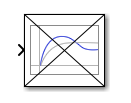In the Simulink model, in the Configuration Parameters, the Model Verification block enabling parameter lets you enable or disable all model verification blocks in a model, regardless of the setting of this option in the block.

#### Programmatic Use

 Parameter: `enabled` Type: character vector Value: `'on'` | `'off'` Default: `'on'`

MATLAB expression to execute when assertion fails.

Because the expression is evaluated in the MATLAB workspace, first define all variables used in the expression in the workspace.

#### Dependencies

To enable this parameter, select Enable assertion.

#### Programmatic Use

 Parameter: `callback` Type: character vector Value: MATLAB expression Default: `''`

Stop the simulation when the input signal violates a bound specified on the Bounds tab during simulation, that is, when assertion fails.

If you run the simulation from a Simulink model window, the Simulation Diagnostics window opens to display an error message. The block where the bound violation occurs is highlighted in the model.

Because selecting this option stops the simulation as soon as the assertion fails, the software does not report assertion failures that might occur later during the simulation. If you want to report all assertion failures, do not select this option.

#### Dependencies

To enable this parameter, select Enable assertion.

#### Programmatic Use

 Parameter: `stopWhenAssertionFail` Type: character vector Value: `'on'` | `'off'` Default: `'off'`

Output a Boolean signal that, at each time step, is:

• True (`1`) if assertion succeeds, that is, the input signal satisfies all bounds

• False (`0`) if assertion fails, that is, the input signal violates a bound

The data type of the output signal is double by default. To set the output data type as Boolean for all blocks that generate logic signals in your Simulink model, select Implement logic signals as Boolean data in Configuration Parameters.

Selecting this parameter adds an output port to the block that you can connect to any block in the model.

Use this parameter to design complex assertion logic. For an example, see Verify Model Using Simulink Control Design and Simulink Verification Blocks (Simulink Control Design).

#### Programmatic Use

 Parameter: `export` Type: character vector Value: `'on'` | `'off'` Default: `'off'`

## Version History

Introduced in R2011b